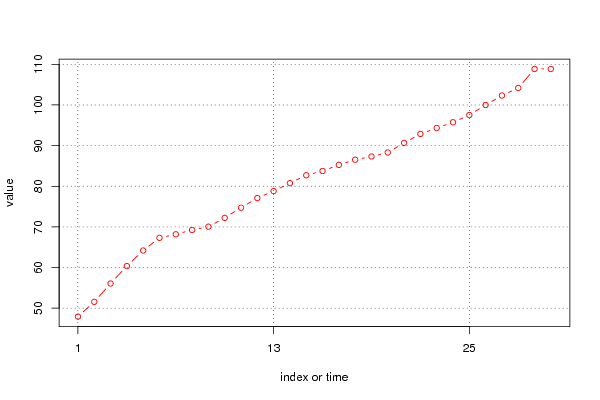## Free Statistics

of Irreproducible Research!

Author's title
Author*The author of this computation has been verified*
R Software Modulerwasp_univariatedataseries.wasp
Title produced by softwareUnivariate Data Series
Date of computationWed, 22 Dec 2010 13:07:15 +0000
Cite this page as followsStatistical Computations at FreeStatistics.org, Office for Research Development and Education, URL https://freestatistics.org/blog/index.php?v=date/2010/Dec/22/t12930234435grqaurjlx4hjky.htm/, Retrieved Sat, 30 Sep 2023 13:26:27 +0000
Statistical Computations at FreeStatistics.org, Office for Research Development and Education, URL https://freestatistics.org/blog/index.php?pk=114190, Retrieved Sat, 30 Sep 2023 13:26:27 +0000
QR Codes:Original text written by user:
IsPrivate?No (this computation is public)
User-defined keywords
Estimated Impact145
Family? (F = Feedback message, R = changed R code, M = changed R Module, P = changed Parameters, D = changed Data)
-     [Univariate Data Series] [Arabica Price in ...] [2008-01-05 23:43:18] [74be16979710d4c4e7c6647856088456]
- RMPD    [Univariate Data Series] [inflatie in Belgi...] [2010-12-22 13:07:15] [03bcd8c83ef1a42b4029a16ba47a4880] [Current]
-    D      [Univariate Data Series] [univariate analyse] [2010-12-22 14:11:03] [30b3e197115d238a51c18bcedc33a6a5]
-   PD      [Univariate Data Series] [univariate analys...] [2010-12-22 14:14:11] [30b3e197115d238a51c18bcedc33a6a5]
-   PD      [Univariate Data Series] [univariate analys...] [2010-12-22 17:44:43] [96348ef82925ade81ab3c243141d80f1]
Feedback Forum

Post a new message
Dataseries X:
47,91
51,56
56,06
60,36
64,19
67,31
68,18
69,24
70,05
72,22
74,72
77,08
78,81
80,78
82,71
83,76
85,26
86,53
87,32
88,31
90,67
92,88
94,33
95,75
97,53
100
102,33
104,19
108,87
108,86


 Summary of computational transaction Raw Input view raw input (R code) Raw Output view raw output of R engine Computing time 1 seconds R Server 'George Udny Yule' @ 72.249.76.132

\begin{tabular}{lllllllll}
\hline
Summary of computational transaction \tabularnewline
Raw Input & view raw input (R code)  \tabularnewline
Raw Output & view raw output of R engine  \tabularnewline
Computing time & 1 seconds \tabularnewline
R Server & 'George Udny Yule' @ 72.249.76.132 \tabularnewline
\hline
\end{tabular}
%Source: https://freestatistics.org/blog/index.php?pk=114190&T=0

[TABLE]
[ROW][C]Summary of computational transaction[/C][/ROW]
[ROW][C]Raw Input[/C][C]view raw input (R code) [/C][/ROW]
[ROW][C]Raw Output[/C][C]view raw output of R engine [/C][/ROW]
[ROW][C]Computing time[/C][C]1 seconds[/C][/ROW]
[ROW][C]R Server[/C][C]'George Udny Yule' @ 72.249.76.132[/C][/ROW]
[/TABLE]
Source: https://freestatistics.org/blog/index.php?pk=114190&T=0

Globally Unique Identifier (entire table): ba.freestatistics.org/blog/index.php?pk=114190&T=0

As an alternative you can also use a QR Code:

The GUIDs for individual cells are displayed in the table below:

 Summary of computational transaction Raw Input view raw input (R code) Raw Output view raw output of R engine Computing time 1 seconds R Server 'George Udny Yule' @ 72.249.76.132

 Univariate Dataseries Name of dataseries inflatie Source Description Number of observations 30

\begin{tabular}{lllllllll}
\hline
Univariate Dataseries \tabularnewline
Name of dataseries & inflatie \tabularnewline
Source &  \tabularnewline
Description &  \tabularnewline
Number of observations & 30 \tabularnewline
\hline
\end{tabular}
%Source: https://freestatistics.org/blog/index.php?pk=114190&T=1

[TABLE]
[ROW][C]Univariate Dataseries[/C][/ROW]
[ROW][C]Name of dataseries[/C][C]inflatie[/C][/ROW]
[ROW][C]Source[/C][C][/C][/ROW]
[ROW][C]Description[/C][C][/C][/ROW]
[ROW][C]Number of observations[/C][C]30[/C][/ROW]
[/TABLE]
Source: https://freestatistics.org/blog/index.php?pk=114190&T=1

Globally Unique Identifier (entire table): ba.freestatistics.org/blog/index.php?pk=114190&T=1

As an alternative you can also use a QR Code:

The GUIDs for individual cells are displayed in the table below:

 Univariate Dataseries Name of dataseries inflatie Source Description Number of observations 30PNG link Postscript link PDF link

Parameters (Session):
par1 = inflatie ; par4 = 12 ;
Parameters (R input):
par1 = inflatie ; par2 = ; par3 = ; par4 = 12 ;
R code (references can be found in the software module):
if (par4 != 'No season') {par4 <- as.numeric(par4)if (par4 < 4) par4 <- 12}summary(x)n <- length(x)bitmap(file='test1.png')if (par4=='No season') {plot(x,col=2,type='b',main=main,xlab=xlab,ylab=ylab,xaxt='n')axis(1,at=seq(1,n,10))}if (par4!='No season') {plot(x,col=2,type='b',main=main,xlab=xlab,ylab=ylab,xaxt='n')axis(1,at=seq(1,n,par4))grid(nx=0,ny=NULL,col='black')abline(v=seq(1,n,par4),col='black',lty='dotted')}dev.off()load(file='createtable')a<-table.start()a<-table.row.start(a)a<-table.element(a,'Univariate Dataseries',2,TRUE)a<-table.row.end(a)a<-table.row.start(a)a<-table.element(a,'Name of dataseries',header=TRUE)a<-table.element(a,par1)a<-table.row.end(a)a<-table.row.start(a)a<-table.element(a,'Source',header=TRUE)a<-table.element(a,par2)a<-table.row.end(a)a<-table.row.start(a)a<-table.element(a,'Description',header=TRUE)a<-table.element(a,par3)a<-table.row.end(a)a<-table.row.start(a)a<-table.element(a,'Number of observations',header=TRUE)a<-table.element(a,length(x))a<-table.row.end(a)a<-table.end(a)table.save(a,file='mytable.tab')